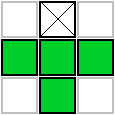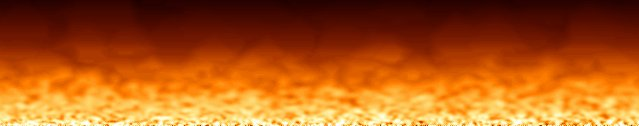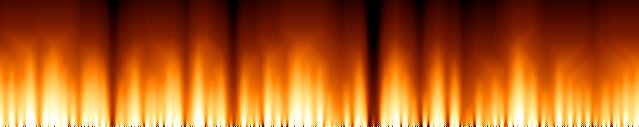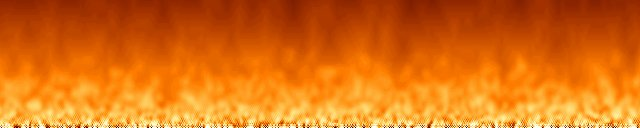# Fire Effect

Back to Index

## Introduction

This is a very short tutorial, about the very classic Fire Effect, and creating a fire color palette for it. Maybe this article will be extended later with fire made by particles or other better looking fires.

## The Classic Fire Effect

This fire effect has been used quite often for java demos, oldskool demos, etc... It's very easy to program, and gives very neat looking fire.

First you have to create a palette, typically of 256 colors, but it can be any other amount as well here because we emulate it. With the HSLtoRGB function it's easy to create a good palette, select a hue range from red to yellow, keep saturation at 255, and brightness from 0 to 255 so that more red colors become darker as well. The palette is created to be used with the drawBuffer function, so the RGB colors are stored in a single integer value in BGR order.

To contain the fire, you need a buffer (called fire[][] here) with the same size as the screen. In a graphics mode that uses a palette, this buffer can be the screen itself, but here we use RGB mode and the palette is emulated. The buffer starts with every pixel at 0. Then at the bottom row, give a random value to each pixel, and keep changing the random values every frame. Each frame, calculate each row of pixels based on the two rows below it: the value of each pixel, becomes the sum of the 3 pixels below it (one directly below it, one to the left of this one, and one to the right of this one), and one pixel directly two rows below it. Then divide the sum through a value slightly larger than 4, so that the fire dies out as it rises. The larger the value you divide it through, the lower the flames can rise. For example, if you use *16, /65, which is the same as dividing through 4.0625, the flames will rise higher than if you use *4, /17, which is the same as dividing through 4.25. If you divide it though 4, the fire keeps rising forever, and if you divide it though 5, it dies out way to fast.

This image shows which pixels are included in the calculation: the pixel with the cross is the current pixel, and the 4 green pixels are the ones used to calculate the value of the current pixel.Do this from top to bottom, if you do the calculations in the wrong order, some pixels will already be recalculated while other pixels that depend on them still have to be calculated. Each new frame may depend only on the values of the previous frame. The top of the screen has y-coordinate 0, and the bottom has y-coordinate h-1.

On the sides of the screen, the pixels have no neighbors to the left or right anymore, and to overcome this, the effect can be made circular so that the rightmost pixel has the leftmost pixel as its neighbor and vica versa. For this, modulo division through the width of the screen can be used on the x-coordinate of the neighbors.

The version of the effect here isn't very fast at high resolutions, but there's still set a maximum number of frames per second by using the waitFrame function, so that it won't run too fast on faster computers in the future.

Because we have a fire buffer, we can use the drawBuffer function instead of drawing each pixel separately, which is much faster. The buffer that gets drawn isn't the same as the fire array though, because that one has values from 0 to 256, which gives the wrong colors. Instead we create a new buffer and store the RGB color gotten from the palette in it, and then draw this buffer (which also has the name "buffer" here).

Put the following code inside the the main.cpp file. The comments in the code will explain a bit more:

 ```#include #include #include #include #include "quickcg.h" using namespace QuickCG; //define the width and height of the screen and the buffers const int screenWidth = 640; const int screenHeight = 128; // Y-coordinate first because we use horizontal scanlines Uint32 fire[screenHeight][screenWidth]; //this buffer will contain the fire Uint32 buffer[screenHeight][screenWidth]; //this is the buffer to be drawn to the screen Uint32 palette; //this will contain the color palette int main(int argc, char *argv[]) { //set up the screen screen(screenWidth, screenHeight, 0, "fire"); //declarations ColorRGB color; //used during palette generation float time = getTime(), oldTime; //the time of this and the previous frame, for timing //make sure the fire buffer is zero in the beginning for(int y = 0; y < h; y++) for(int x = 0; x < w; x++) fire[y][x] = 0; //generate the palette for(int x = 0; x < 256; x++) { //HSLtoRGB is used to generate colors: //Hue goes from 0 to 85: red to yellow //Saturation is always the maximum: 255 //Lightness is 0..255 for x=0..128, and 255 for x=128..255 color = HSLtoRGB(ColorHSL(x / 3, 255, std::min(255, x * 2))); //set the palette to the calculated RGB value palette[x] = RGBtoINT(color); } //start the loop (one frame per loop) while(!done()) { //timing: set to maximum 50 milliseconds per frame = 20 frames per second oldTime = time; waitFrame(oldTime, 0.05); time = getTime(); //randomize the bottom row of the fire buffer for(int x = 0; x < w; x++) fire[h - 1][x] = abs(32768 + rand()) % 256; //do the fire calculations for every pixel, from top to bottom for(int y = 0; y < h - 1; y++) for(int x = 0; x < w; x++) { fire[y][x] = ((fire[(y + 1) % h][(x - 1 + w) % w] + fire[(y + 1) % h][(x) % w] + fire[(y + 1) % h][(x + 1) % w] + fire[(y + 2) % h][(x) % w]) * 32) / 129; } //set the drawing buffer to the fire buffer, using the palette colors for(int y = 0; y < h; y++) for(int x = 0; x < w; x++) { buffer[y][x] = palette[fire[y][x]]; } //draw the buffer and redraw the screen drawBuffer(buffer); redraw(); } } ```

If you run it, first everything is black, and the flames rise two pixels every frame until they reached their maximum height. Then they'll still keep animating because the bottom is randomized every frame:If you don't randomize the bottom of the fire buffer every frame but only at the beginning, the flames won't be animated either, and once they have risen to their maximum height, they'll stay still forever:By changing parameters, you can tweak the fire, for example this is what I got for changing the calculations to:

 ``` fire[y][x] = ((fire[(y + 1) % h][(x - 1 + w) % w] + fire[(y + 2) % h][(x) % w] + fire[(y + 1) % h][(x + 1) % w] + fire[(y + 3) % h][(x) % w]) * 64) / 257; ```Instead of randomizing the bottom row of pixels you can also randomize the edges of objects, for example to make flaming text, ...

Last edited: 20 august 2007